# Even-Hole-Free Graphs

A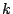-division of a graph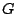is a partition of its vertex-set into sets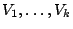such that no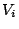contains a largest clique of. A graph is-divisible if each of its induced subgraphs with at least one edge has a-division.

Conforti, Cornuéjols, Kapoor and Vuškovic designed a polynomial algorithm to recognize even-hole-free graphs.

Conjecture 2 (Hoàng). Every even-hole-free graph is 3-divisible.

Conjecture 3 (Hoàng). Ifis an even-hole-free graph then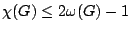.

Hayward and Reed proposed the following.

Conjecture 4 (Hayward and Reed). An even-hole-free graph contains a vertex whose neighbourhood can be partitioned into two cliques.

Conjecture 4 implies Conjecture 3 which in turn implies Conjecture 2. Letbe an even-hole-free graph. Conjecture 4 implies that each induced subgraph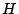ofhas a vertex of degree at most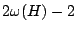, and therefore. Since any graph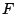is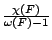-divisible,is 3-divisible.

Contributed by Chinh Hoang.

Back to the main index for Perfect Graphs.